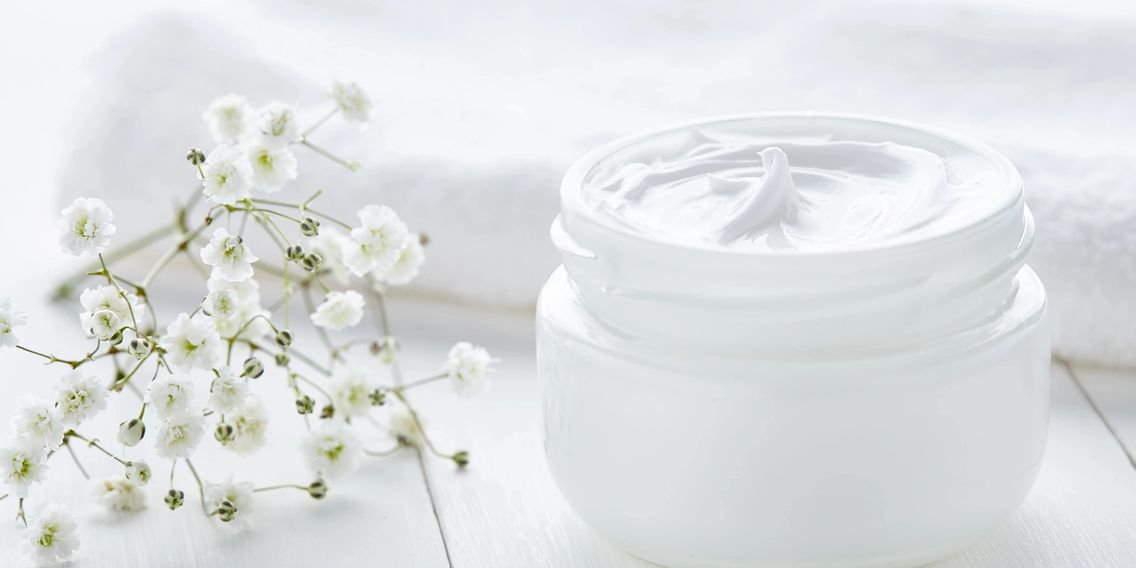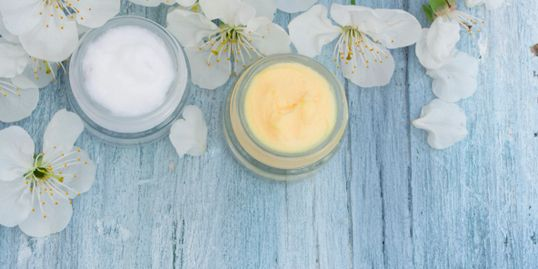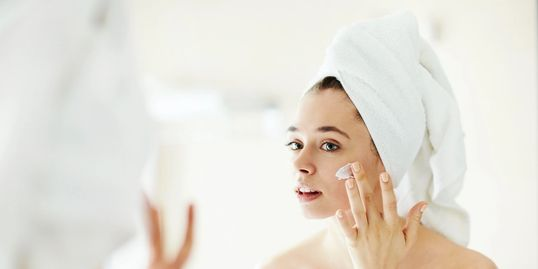N-E-L N-E-L N-E-L N-E-L N-E-L N-E-L N-E-L N-E-L N-E-L N-E-L N-E-L N-E-L N-E-L N-E-L N-E-L N-E-L N-E-L N-E-L N-E-L N-E-L N-E-L N-E-L N-E-L N-E-L N-E-L N-E-L N-E-L N-E-L N-E-L N-E-L N-E-L N-E-L N-E-L N-E-L N-E-L N-E-L N-E-L N-E-L N-E-L N-E-L N-E-L N-E-L N-E-L N-E-L N-E-L N-E-L N-E-L N-E-L N-E-L N-E-L N-E-L N-E-L N-E-L N-E-L N-E-L N-E-L N-E-L N-E-L N-E-L N-E-L N-E-L N-E-L N-E-L N-E-L N-E-L N-E-L N-E-L N-E-L N-E-L N-E-L N-E-L N-E-L N-E-L N-E-L N-E-L N-E-L N-E-L N-E-L N-E-L N-E-L N-E-L N-E-L N-E-L N-E-L N-E-L N-E-L N-E-L N-E-L N-E-L N-E-L N-E-L N-E-L N-E-L N-E-L N-E-L N-E-L N-E-L N-E-L N-E-L N-E-L N-E-L N-E-L N-E-L N-E-L N-E-L N-E-L N-E-L N-E-L N-E-L N-E-L N-E-L N-E-L N-E-L N-E-L N-E-L N-E-L N-E-L N-E-L N-E-L N-E-L N-E-L N-E-L N-E-L N-E-L N-E-L N-E-L N-E-L N-E-L N-E-L N-E-L N-E-L N-E-L N-E-L N-E-L N-E-L N-E-L N-E-L N-E-L N-E-L N-E-L N-E-L N-E-L N-E-L N-E-L N-E-L N-E-L N-E-L N-E-L N-E-L N-E-L N-E-L N-E-L N-E-L N-E-L N-E-L N-E-L N-E-L N-E-L N-E-L N-E-L N-E-L N-E-L N-E-L N-E-L N-E-L N-E-L N-E-L N-E-L N-E-L N-E-L N-E-L N-E-L N-E-L N-E-L N-E-L N-E-L N-E-L N-E-L N-E-L N-E-L N-E-L N-E-L N-E-L N-E-L N-E-L N-E-L N-E-L N-E-L N-E-L N-E-L N-E-L N-E-L N-E-L N-E-L N-E-L N-E-L N-E-L N-E-L N-E-L N-E-L N-E-L N-E-L N-E-L N-E-L N-E-L N-E-L N-E-L N-E-L N-E-L N-E-L N-E-L N-E-L N-E-L N-E-L N-E-L N-E-L N-E-L N-E-L N-E-L N-E-L N-E-L N-E-L N-E-L N-E-L N-E-L N-E-L N-E-L N-E-L N-E-L N-E-L N-E-L N-E-L N-E-L N-E-L N-E-L N-E-L N-E-L N-E-L N-E-L N-E-L N-E-L N-E-L N-E-L N-E-L N-E-L N-E-L N-E-L N-E-L N-E-L N-E-L N-E-L N-E-L N-E-L N-E-L N-E-L N-E-L N-E-L N-E-L N-E-L N-E-L N-E-L N-E-L N-E-L N-E-L N-E-L N-E-L N-E-L

# Making Beauty Personal

Helping you find beautiful!

N-E-L N-E-L N-E-L N-E-L N-E-L N-E-L N-E-L N-E-L N-E-L N-E-L N-E-L N-E-L N-E-L N-E-L N-E-L N-E-L N-E-L N-E-L N-E-L N-E-L N-E-L N-E-L N-E-L N-E-L N-E-L N-E-L N-E-L N-E-L N-E-L N-E-L N-E-L N-E-L N-E-L N-E-L N-E-L N-E-L N-E-L N-E-L N-E-L N-E-L N-E-L N-E-L N-E-L N-E-L N-E-L N-E-L N-E-L N-E-L N-E-L N-E-L N-E-L N-E-L N-E-L N-E-L N-E-L N-E-L N-E-L N-E-L N-E-L N-E-L N-E-L N-E-L N-E-L N-E-L N-E-L N-E-L N-E-L N-E-L N-E-L N-E-L N-E-L N-E-L N-E-L N-E-L N-E-L N-E-L N-E-L N-E-L N-E-L N-E-L N-E-L N-E-L N-E-L N-E-L N-E-L N-E-L N-E-L N-E-L N-E-L N-E-L N-E-L N-E-L N-E-L N-E-L N-E-L N-E-L N-E-L N-E-L N-E-L N-E-L N-E-L N-E-L N-E-L N-E-L N-E-L N-E-L N-E-L N-E-L N-E-L N-E-L N-E-L N-E-L N-E-L N-E-L N-E-L N-E-L N-E-L N-E-L N-E-L N-E-L N-E-L N-E-L N-E-L N-E-L N-E-L N-E-L N-E-L N-E-L N-E-L N-E-L N-E-L N-E-L N-E-L N-E-L N-E-L N-E-L N-E-L N-E-L N-E-L N-E-L N-E-L N-E-L N-E-L N-E-L N-E-L N-E-L N-E-L N-E-L N-E-L N-E-L N-E-L N-E-L N-E-L N-E-L N-E-L N-E-L N-E-L N-E-L N-E-L N-E-L N-E-L N-E-L N-E-L N-E-L N-E-L N-E-L N-E-L N-E-L N-E-L N-E-L N-E-L N-E-L N-E-L N-E-L N-E-L N-E-L N-E-L N-E-L N-E-L N-E-L N-E-L N-E-L N-E-L N-E-L N-E-L N-E-L N-E-L N-E-L N-E-L N-E-L N-E-L N-E-L N-E-L N-E-L N-E-L N-E-L N-E-L N-E-L N-E-L N-E-L N-E-L N-E-L N-E-L N-E-L N-E-L N-E-L N-E-L N-E-L N-E-L N-E-L N-E-L N-E-L N-E-L N-E-L N-E-L N-E-L N-E-L N-E-L N-E-L N-E-L N-E-L N-E-L N-E-L N-E-L N-E-L N-E-L N-E-L N-E-L N-E-L N-E-L N-E-L N-E-L N-E-L N-E-L N-E-L N-E-L N-E-L N-E-L N-E-L N-E-L N-E-L N-E-L N-E-L N-E-L N-E-L N-E-L N-E-L N-E-L N-E-L N-E-L N-E-L N-E-L N-E-L N-E-L N-E-L N-E-L N-E-L N-E-L N-E-L N-E-L N-E-L N-E-L N-E-L N-E-L N-E-L N-E-L N-E-L

# Making Beauty Personal

Helping you find beautiful!

## Established in 2015

#### Committed to QualityQuality should never be a compromise. From France's most popular brand, we want to find it and bring it to you so you can look great.

#### 100% Satisfaction GuaranteedWe want you to be completely happy with your shopping experience from order to delivery. If you have questions about our products, delivery, or shipping, get in touch!

#### Where to buy our productsWe distribute Mary Cohr to a large variety of Salons within South Africa and Swaziland, Contact us to find a salon in your neighbourhood.

### Subscribe

Be the first to hear about upcoming sales, special discounts, and hot beauty trends.